# Plotting¶

## Introduction¶

Labeled data enables expressive computations. These same labels can also be used to easily create informative plots.

xarray’s plotting capabilities are centered around xarray.DataArray objects. To plot xarray.Dataset objects simply access the relevant DataArrays, ie dset['var1']. Here we focus mostly on arrays 2d or larger. If your data fits nicely into a pandas DataFrame then you’re better off using one of the more developed tools there.

xarray plotting functionality is a thin wrapper around the popular matplotlib library. Matplotlib syntax and function names were copied as much as possible, which makes for an easy transition between the two. Matplotlib must be installed before xarray can plot.

For more extensive plotting applications consider the following projects:

• Seaborn: “provides a high-level interface for drawing attractive statistical graphics.” Integrates well with pandas.
• HoloViews and GeoViews: “Composable, declarative data structures for building even complex visualizations easily.” Includes native support for xarray objects.
• Cartopy: Provides cartographic tools.

### Imports¶

The following imports are necessary for all of the examples.

In : import numpy as np

In : import pandas as pd

In : import matplotlib.pyplot as plt

In : import xarray as xr


For these examples we’ll use the North American air temperature dataset.

In : airtemps = xr.tutorial.load_dataset('air_temperature')

In : airtemps
Out:
<xarray.Dataset>
Dimensions:  (lat: 25, lon: 53, time: 2920)
Coordinates:
* lat      (lat) float32 75.0 72.5 70.0 67.5 65.0 62.5 60.0 57.5 55.0 52.5 ...
* lon      (lon) float32 200.0 202.5 205.0 207.5 210.0 212.5 215.0 217.5 ...
* time     (time) datetime64[ns] 2013-01-01 2013-01-01T06:00:00 ...
Data variables:
air      (time, lat, lon) float32 241.2 242.5 243.5 244.0 244.1 243.89 ...
Attributes:
Conventions:  COARDS
title:        4x daily NMC reanalysis (1948)
description:  Data is from NMC initialized reanalysis\n(4x/day).  These a...
platform:     Model
references:   http://www.esrl.noaa.gov/psd/data/gridded/data.ncep.reanaly...

# Convert to celsius
In : air = airtemps.air - 273.15


## One Dimension¶

### Simple Example¶

xarray uses the coordinate name to label the x axis.

In : air1d = air.isel(lat=10, lon=10)

In : air1d.plot()
Out: [<matplotlib.lines.Line2D at 0x7fa54537b2e8>]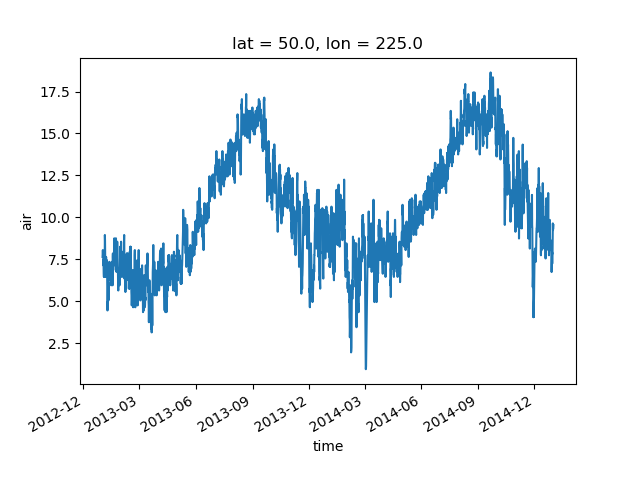Additional arguments are passed directly to the matplotlib function which does the work. For example, xarray.plot.line() calls matplotlib.pyplot.plot passing in the index and the array values as x and y, respectively. So to make a line plot with blue triangles a matplotlib format string can be used:

In : air1d[:200].plot.line('b-^')
Out: [<matplotlib.lines.Line2D at 0x7fa548f52438>]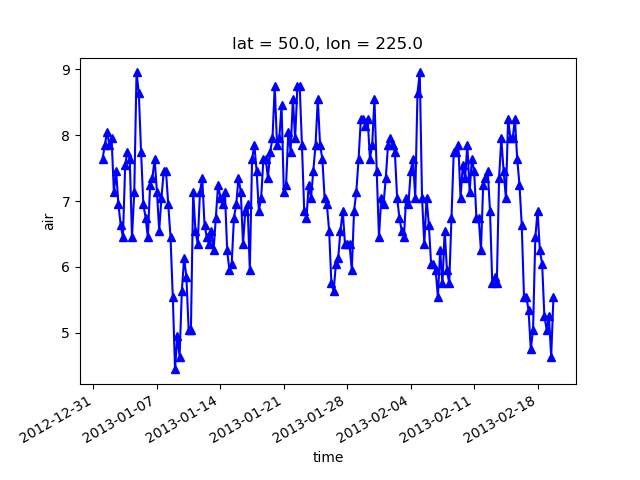Note

Not all xarray plotting methods support passing positional arguments to the wrapped matplotlib functions, but they do all support keyword arguments.

Keyword arguments work the same way, and are more explicit.

In : air1d[:200].plot.line(color='purple', marker='o')
Out: [<matplotlib.lines.Line2D at 0x7fa54550f7b8>]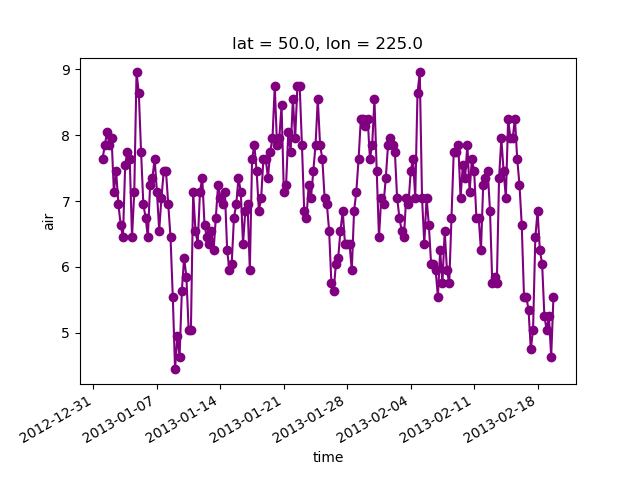### Adding to Existing Axis¶

To add the plot to an existing axis pass in the axis as a keyword argument ax. This works for all xarray plotting methods. In this example axes is an array consisting of the left and right axes created by plt.subplots.

In : fig, axes = plt.subplots(ncols=2)

In : axes
Out:
array([<matplotlib.axes._subplots.AxesSubplot object at 0x7fa54ca737f0>,
<matplotlib.axes._subplots.AxesSubplot object at 0x7fa56595bb38>], dtype=object)

In : air1d.plot(ax=axes)
Out: [<matplotlib.lines.Line2D at 0x7fa54d42c198>]

In : air1d.plot.hist(ax=axes)
Out:
(array([   9.,   38.,  255.,  584.,  542.,  489.,  368.,  258.,  327.,   50.]),
array([  0.95 ,   2.719,   4.488, ...,  15.102,  16.871,  18.64 ]),
<a list of 10 Patch objects>)

In : plt.tight_layout()

In : plt.show()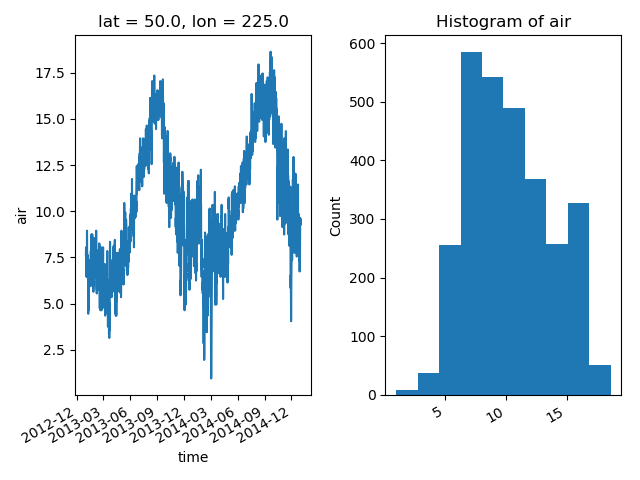On the right is a histogram created by xarray.plot.hist().

### Controlling the figure size¶

You can pass a figsize argument to all xarray’s plotting methods to control the figure size. For convenience, xarray’s plotting methods also support the aspect and size arguments which control the size of the resulting image via the formula figsize = (aspect * size, size):

In : air1d.plot(aspect=2, size=3)
Out: [<matplotlib.lines.Line2D at 0x7fa5455622e8>]

In : plt.tight_layout()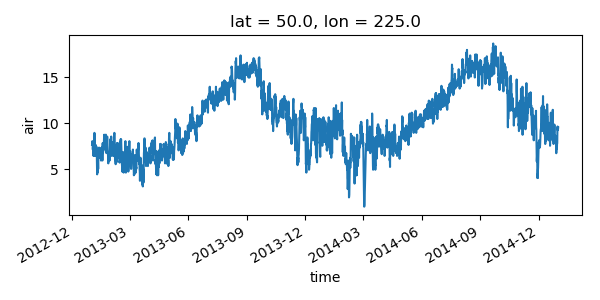This feature also works with Faceting. For facet plots, size and aspect refer to a single panel (so that aspect * size gives the width of each facet in inches), while figsize refers to the entire figure (as for matplotlib’s figsize argument).

Note

If figsize or size are used, a new figure is created, so this is mutually exclusive with the ax argument.

Note

The convention used by xarray (figsize = (aspect * size, size)) is borrowed from seaborn: it is therefore not equivalent to matplotlib’s.

### Multiple lines showing variation along a dimension¶

It is possible to make line plots of two-dimensional data by calling xarray.plot.line() with appropriate arguments. Consider the 3D variable air defined above. We can use line plots to check the variation of air temperature at three different latitudes along a longitude line:

In : air.isel(lon=10, lat=[19,21,22]).plot.line(x='time')
Out:
[<matplotlib.lines.Line2D at 0x7fa54d396898>,
<matplotlib.lines.Line2D at 0x7fa545533630>,
<matplotlib.lines.Line2D at 0x7fa5455333c8>]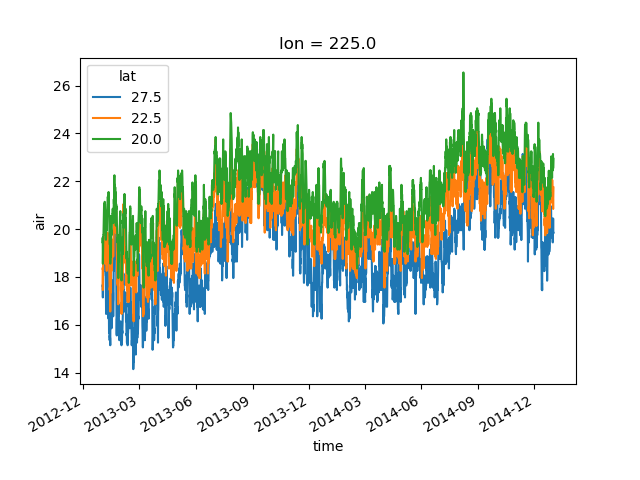It is required to explicitly specify either

1. x: the dimension to be used for the x-axis, or
2. hue: the dimension you want to represent by multiple lines.

Thus, we could have made the previous plot by specifying hue='lat' instead of x='time'. If required, the automatic legend can be turned off using add_legend=False.

### Dimension along y-axis¶

It is also possible to make line plots such that the data are on the x-axis and a dimension is on the y-axis. This can be done by specifying the appropriate y keyword argument.

In : air.isel(time=10, lon=[10, 11]).plot.line(y='lat', hue='lon')
Out:
[<matplotlib.lines.Line2D at 0x7fa544d4b550>,
<matplotlib.lines.Line2D at 0x7fa544d4b3c8>]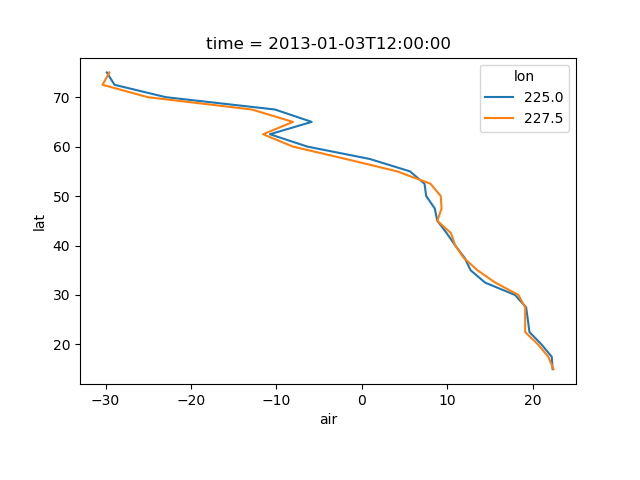## Two Dimensions¶

### Simple Example¶

The default method xarray.DataArray.plot() calls xarray.plot.pcolormesh() by default when the data is two-dimensional.

In : air2d = air.isel(time=500)

In : air2d.plot()
Out: <matplotlib.collections.QuadMesh at 0x7fa5442d6d68>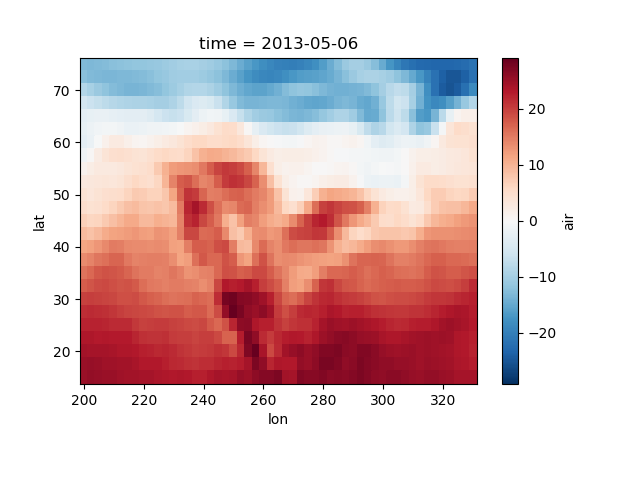All 2d plots in xarray allow the use of the keyword arguments yincrease and xincrease.

In : air2d.plot(yincrease=False)
Out: <matplotlib.collections.QuadMesh at 0x7fa544225eb8>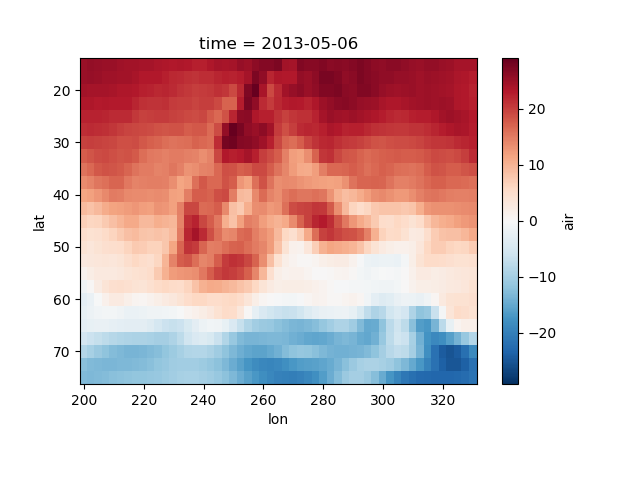Note

We use xarray.plot.pcolormesh() as the default two-dimensional plot method because it is more flexible than xarray.plot.imshow(). However, for large arrays, imshow can be much faster than pcolormesh. If speed is important to you and you are plotting a regular mesh, consider using imshow.

### Missing Values¶

xarray plots data with Missing values.

In : bad_air2d = air2d.copy()

In : bad_air2d[dict(lat=slice(0, 10), lon=slice(0, 25))] = np.nan

Out: <matplotlib.collections.QuadMesh at 0x7fa544205588>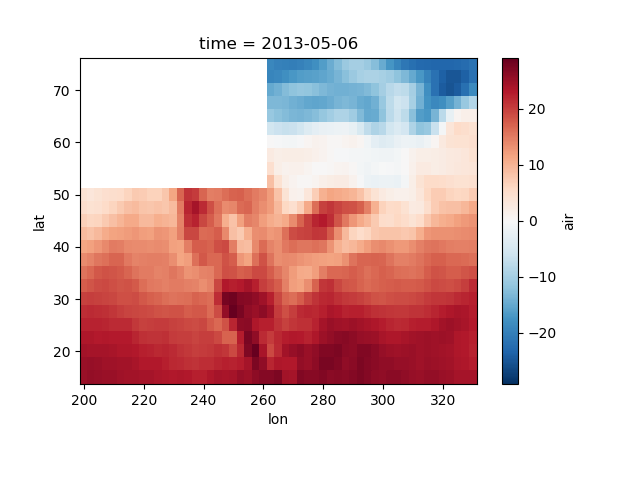### Nonuniform Coordinates¶

It’s not necessary for the coordinates to be evenly spaced. Both xarray.plot.pcolormesh() (default) and xarray.plot.contourf() can produce plots with nonuniform coordinates.

In : b = air2d.copy()

# Apply a nonlinear transformation to one of the coords
In : b.coords['lat'] = np.log(b.coords['lat'])

In : b.plot()
Out: <matplotlib.collections.QuadMesh at 0x7fa544161c50>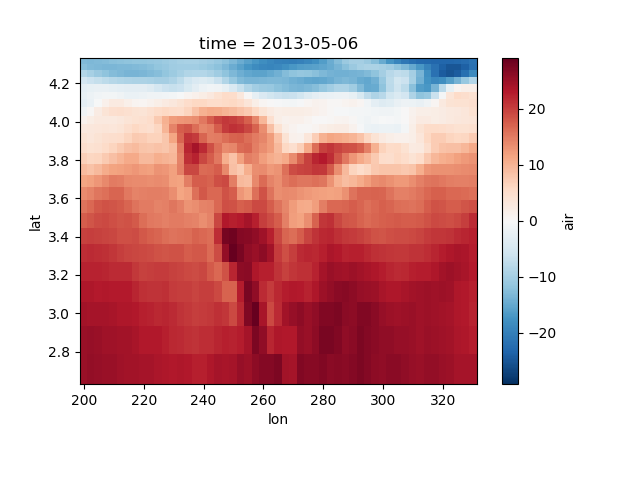### Calling Matplotlib¶

Since this is a thin wrapper around matplotlib, all the functionality of matplotlib is available.

In : air2d.plot(cmap=plt.cm.Blues)
Out: <matplotlib.collections.QuadMesh at 0x7fa544150390>

In : plt.title('These colors prove North America\nhas fallen in the ocean')
Out: Text(0.5,1,'These colors prove North America\nhas fallen in the ocean')

In : plt.ylabel('latitude')
Out: Text(0,0.5,'latitude')

In : plt.xlabel('longitude')
Out: Text(0.5,0,'longitude')

In : plt.tight_layout()

In : plt.show()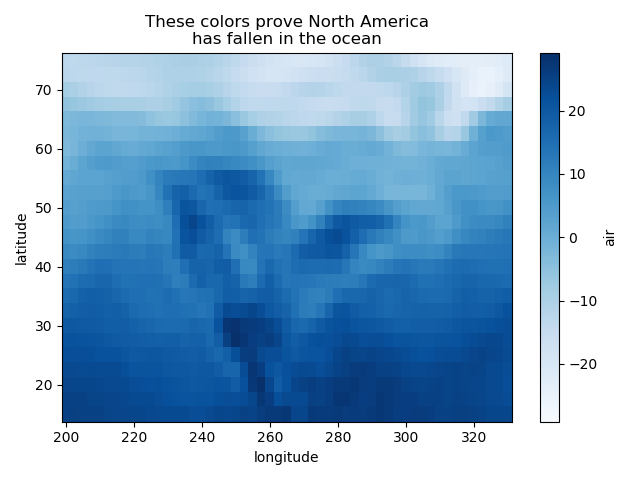Note

xarray methods update label information and generally play around with the axes. So any kind of updates to the plot should be done after the call to the xarray’s plot. In the example below, plt.xlabel effectively does nothing, since d_ylog.plot() updates the xlabel.

In : plt.xlabel('Never gonna see this.')
Out: Text(0.5,0,'Never gonna see this.')

In : air2d.plot()
Out: <matplotlib.collections.QuadMesh at 0x7fa5442c37f0>

In : plt.show()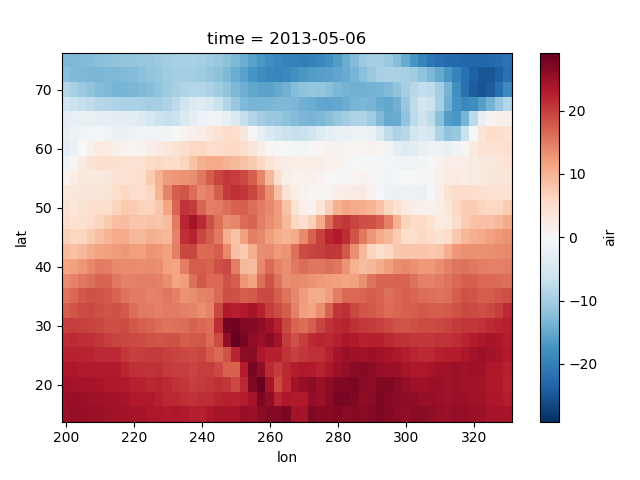### Colormaps¶

xarray borrows logic from Seaborn to infer what kind of color map to use. For example, consider the original data in Kelvins rather than Celsius:

In : airtemps.air.isel(time=0).plot()
Out: <matplotlib.collections.QuadMesh at 0x7fa5454996a0>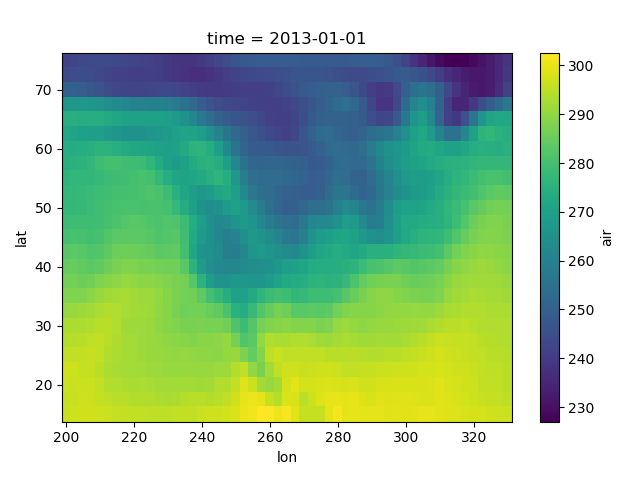The Celsius data contain 0, so a diverging color map was used. The Kelvins do not have 0, so the default color map was used.

### Robust¶

Outliers often have an extreme effect on the output of the plot. Here we add two bad data points. This affects the color scale, washing out the plot.

In : air_outliers = airtemps.air.isel(time=0).copy()

In : air_outliers[0, 0] = 100

In : air_outliers[-1, -1] = 400

In : air_outliers.plot()
Out: <matplotlib.collections.QuadMesh at 0x7fa544172da0>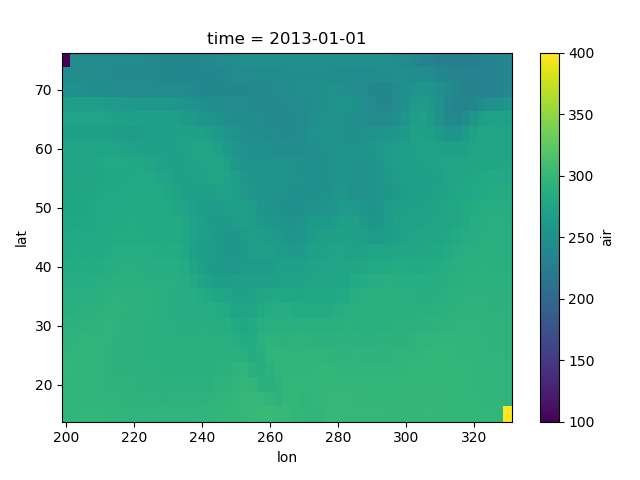This plot shows that we have outliers. The easy way to visualize the data without the outliers is to pass the parameter robust=True. This will use the 2nd and 98th percentiles of the data to compute the color limits.

In : air_outliers.plot(robust=True)
Out: <matplotlib.collections.QuadMesh at 0x7fa52ffc74e0>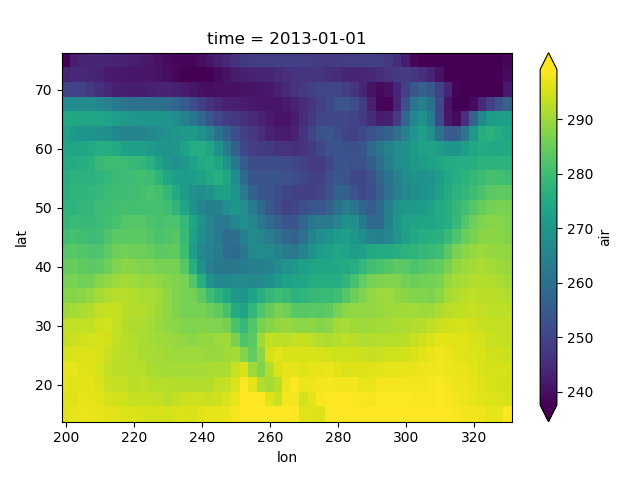Observe that the ranges of the color bar have changed. The arrows on the color bar indicate that the colors include data points outside the bounds.

### Discrete Colormaps¶

It is often useful, when visualizing 2d data, to use a discrete colormap, rather than the default continuous colormaps that matplotlib uses. The levels keyword argument can be used to generate plots with discrete colormaps. For example, to make a plot with 8 discrete color intervals:

In : air2d.plot(levels=8)
Out: <matplotlib.collections.QuadMesh at 0x7fa52ff59080>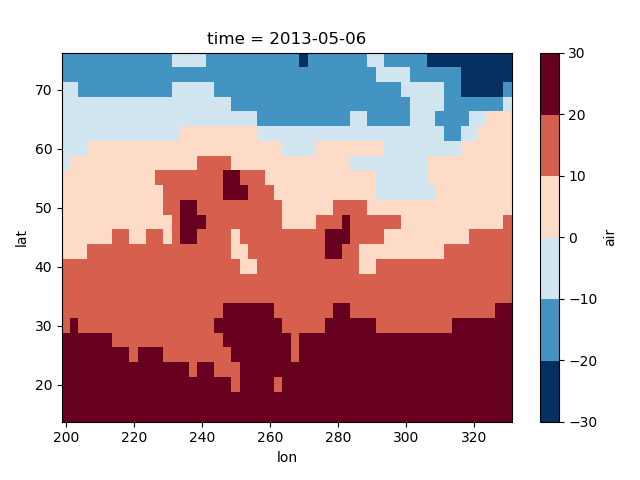It is also possible to use a list of levels to specify the boundaries of the discrete colormap:

In : air2d.plot(levels=[0, 12, 18, 30])
Out: <matplotlib.collections.QuadMesh at 0x7fa52fed2438>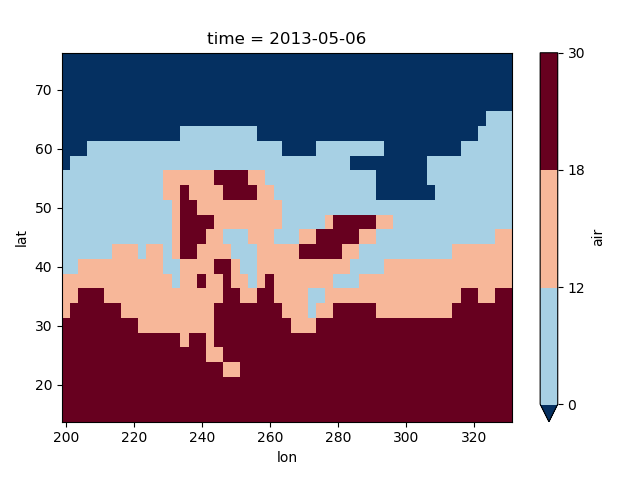You can also specify a list of discrete colors through the colors argument:

In : flatui = ["#9b59b6", "#3498db", "#95a5a6", "#e74c3c", "#34495e", "#2ecc71"]

In : air2d.plot(levels=[0, 12, 18, 30], colors=flatui)
Out: <matplotlib.collections.QuadMesh at 0x7fa52fe4a390>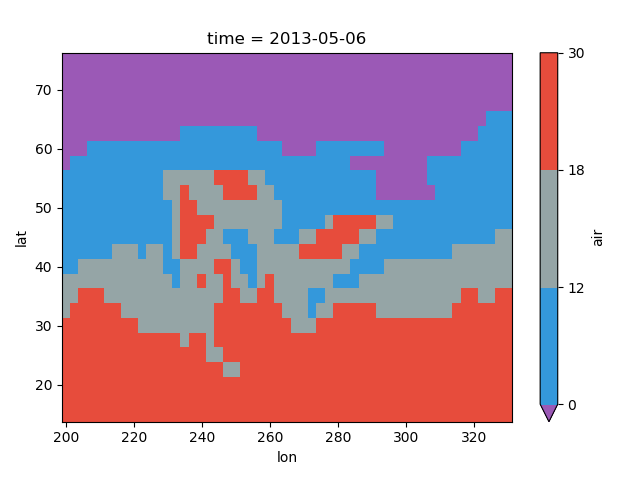Finally, if you have Seaborn installed, you can also specify a seaborn color palette to the cmap argument. Note that levels must be specified with seaborn color palettes if using imshow or pcolormesh (but not with contour or contourf, since levels are chosen automatically).

In : air2d.plot(levels=10, cmap='husl')
Out: <matplotlib.collections.QuadMesh at 0x7fa52fe3a5f8>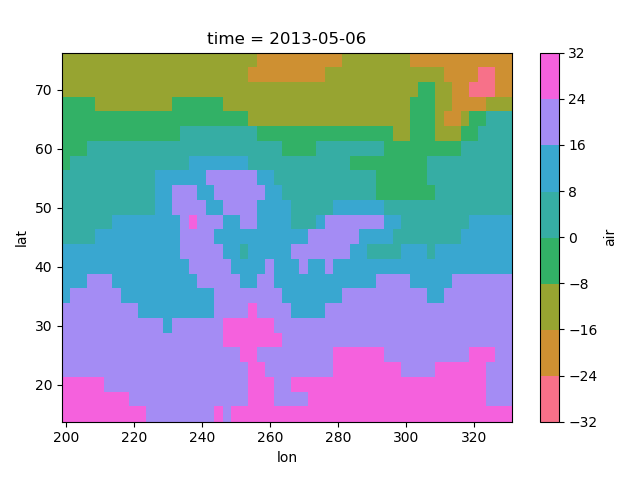## Faceting¶

Faceting here refers to splitting an array along one or two dimensions and plotting each group. xarray’s basic plotting is useful for plotting two dimensional arrays. What about three or four dimensional arrays? That’s where facets become helpful.

Consider the temperature data set. There are 4 observations per day for two years which makes for 2920 values along the time dimension. One way to visualize this data is to make a seperate plot for each time period.

The faceted dimension should not have too many values; faceting on the time dimension will produce 2920 plots. That’s too much to be helpful. To handle this situation try performing an operation that reduces the size of the data in some way. For example, we could compute the average air temperature for each month and reduce the size of this dimension from 2920 -> 12. A simpler way is to just take a slice on that dimension. So let’s use a slice to pick 6 times throughout the first year.

In : t = air.isel(time=slice(0, 365 * 4, 250))

In : t.coords
Out:
Coordinates:
* lat      (lat) float32 75.0 72.5 70.0 67.5 65.0 62.5 60.0 57.5 55.0 52.5 ...
* lon      (lon) float32 200.0 202.5 205.0 207.5 210.0 212.5 215.0 217.5 ...
* time     (time) datetime64[ns] 2013-01-01 2013-03-04T12:00:00 2013-05-06 ...


### Simple Example¶

The easiest way to create faceted plots is to pass in row or col arguments to the xarray plotting methods/functions. This returns a xarray.plot.FacetGrid object.

In : g_simple = t.plot(x='lon', y='lat', col='time', col_wrap=3)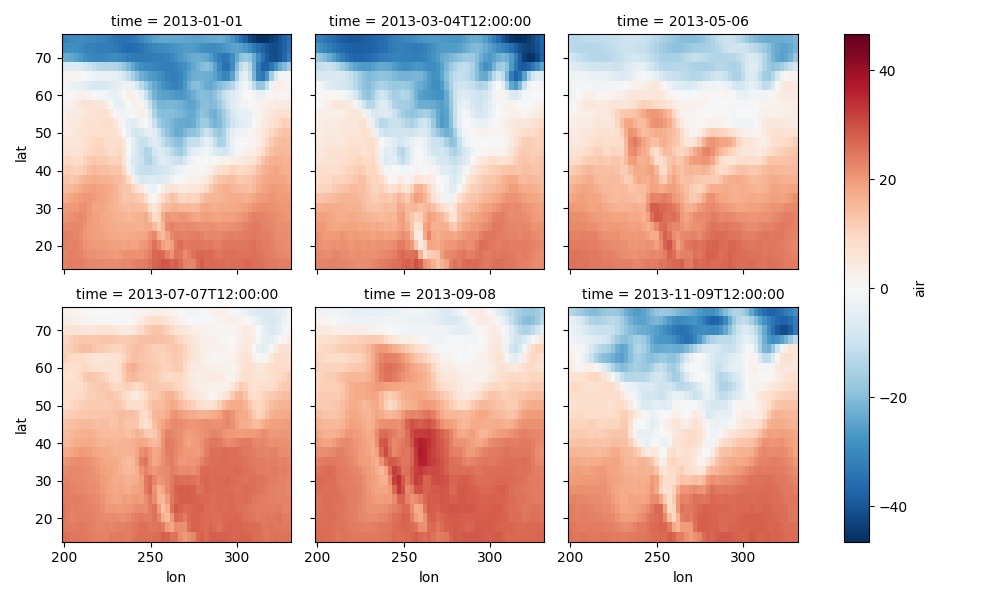### 4 dimensional¶

For 4 dimensional arrays we can use the rows and columns of the grids. Here we create a 4 dimensional array by taking the original data and adding a fixed amount. Now we can see how the temperature maps would compare if one were much hotter.

In : t2 = t.isel(time=slice(0, 2))

In : t4d = xr.concat([t2, t2 + 40], pd.Index(['normal', 'hot'], name='fourth_dim'))

# This is a 4d array
In : t4d.coords
Out:
Coordinates:
* lat         (lat) float32 75.0 72.5 70.0 67.5 65.0 62.5 60.0 57.5 55.0 ...
* lon         (lon) float32 200.0 202.5 205.0 207.5 210.0 212.5 215.0 ...
* time        (time) datetime64[ns] 2013-01-01 2013-03-04T12:00:00
* fourth_dim  (fourth_dim) object 'normal' 'hot'

In : t4d.plot(x='lon', y='lat', col='time', row='fourth_dim')
Out: <xarray.plot.facetgrid.FacetGrid at 0x7fa52fcea588>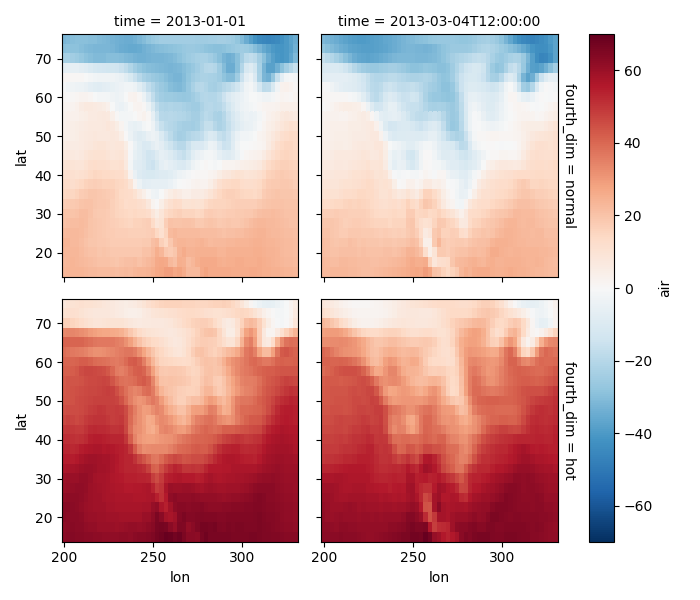### Other features¶

Faceted plotting supports other arguments common to xarray 2d plots.

In : hasoutliers = t.isel(time=slice(0, 5)).copy()

In : hasoutliers[0, 0, 0] = -100

In : hasoutliers[-1, -1, -1] = 400

In : g = hasoutliers.plot.pcolormesh('lon', 'lat', col='time', col_wrap=3,
....:                                 robust=True, cmap='viridis')
....: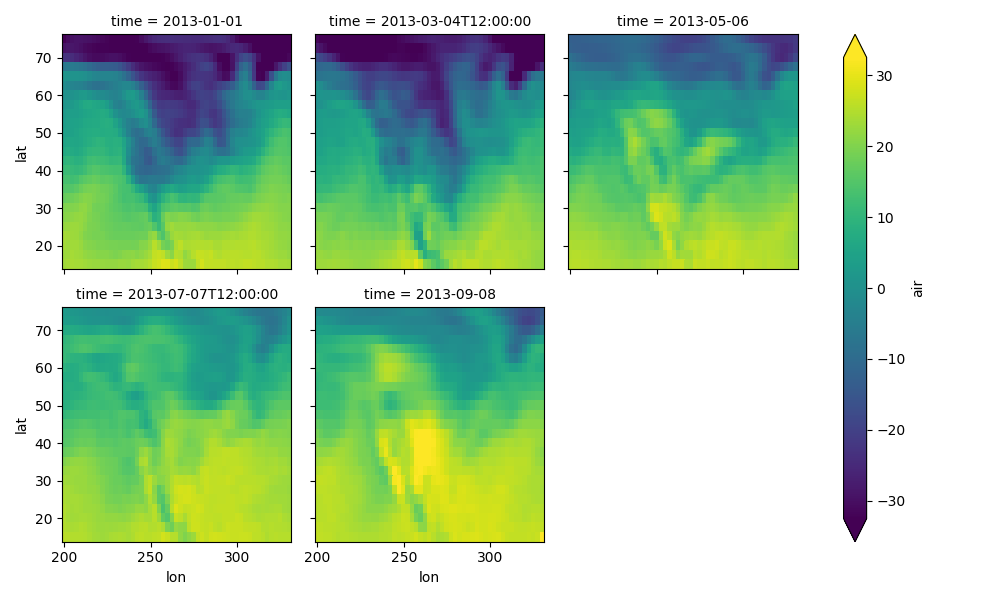### FacetGrid Objects¶

xarray.plot.FacetGrid is used to control the behavior of the multiple plots. It borrows an API and code from Seaborn’s FacetGrid. The structure is contained within the axes and name_dicts attributes, both 2d Numpy object arrays.

In : g.axes
Out:
array([[<matplotlib.axes._subplots.AxesSubplot object at 0x7fa52fa870b8>,
<matplotlib.axes._subplots.AxesSubplot object at 0x7fa52fa5e2e8>,
<matplotlib.axes._subplots.AxesSubplot object at 0x7fa52fa15be0>],
[<matplotlib.axes._subplots.AxesSubplot object at 0x7fa52f9d15f8>,
<matplotlib.axes._subplots.AxesSubplot object at 0x7fa52f987278>,
<matplotlib.axes._subplots.AxesSubplot object at 0x7fa52faf10b8>]], dtype=object)

In : g.name_dicts
Out:
array([[{'time': numpy.datetime64('2013-01-01T00:00:00.000000000')},
{'time': numpy.datetime64('2013-03-04T12:00:00.000000000')},
{'time': numpy.datetime64('2013-05-06T00:00:00.000000000')}],
[{'time': numpy.datetime64('2013-07-07T12:00:00.000000000')},
{'time': numpy.datetime64('2013-09-08T00:00:00.000000000')}, None]], dtype=object)


It’s possible to select the xarray.DataArray or xarray.Dataset corresponding to the FacetGrid through the name_dicts.

In : g.data.loc[g.name_dicts[0, 0]]
Out:
<xarray.DataArray 'air' (lat: 25, lon: 53)>
array([[-100.      ,  -30.649994,  -29.649994, ...,  -40.350006,  -37.649994,
-34.550003],
[ -29.350006,  -28.649994,  -28.449997, ...,  -40.350006,  -37.850006,
-33.850006],
[ -23.149994,  -23.350006,  -24.259995, ...,  -39.949997,  -36.759995,
-31.449997],
...,
[  23.450012,   23.049988,   23.25    , ...,   22.25    ,   21.950012,
21.549988],
[  22.75    ,   23.049988,   23.640015, ...,   22.75    ,   22.75    ,
22.049988],
[  23.140015,   23.640015,   23.950012, ...,   23.75    ,   23.640015,
23.450012]], dtype=float32)
Coordinates:
* lat      (lat) float32 75.0 72.5 70.0 67.5 65.0 62.5 60.0 57.5 55.0 52.5 ...
* lon      (lon) float32 200.0 202.5 205.0 207.5 210.0 212.5 215.0 217.5 ...
time     datetime64[ns] 2013-01-01


Here is an example of using the lower level API and then modifying the axes after they have been plotted.

In : g = t.plot.imshow('lon', 'lat', col='time', col_wrap=3, robust=True)

In : for i, ax in enumerate(g.axes.flat):
....:     ax.set_title('Air Temperature %d' % i)
....:

In : bottomright = g.axes[-1, -1]

In : bottomright.annotate('bottom right', (240, 40))
Out: Text(240,40,'bottom right')

In : plt.show()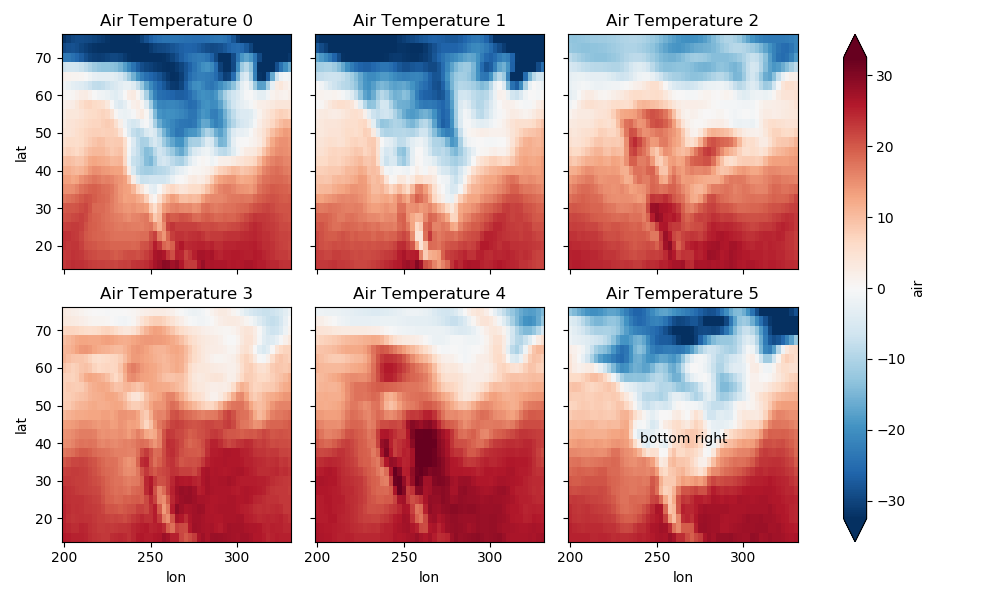TODO: add an example of using the map method to plot dataset variables (e.g., with plt.quiver).

## Maps¶

To follow this section you’ll need to have Cartopy installed and working.

This script will plot the air temperature on a map.

In : import cartopy.crs as ccrs

In : air = xr.tutorial.load_dataset('air_temperature').air

In : ax = plt.axes(projection=ccrs.Orthographic(-80, 35))

In : air.isel(time=0).plot.contourf(ax=ax, transform=ccrs.PlateCarree());

In : ax.set_global(); ax.coastlines();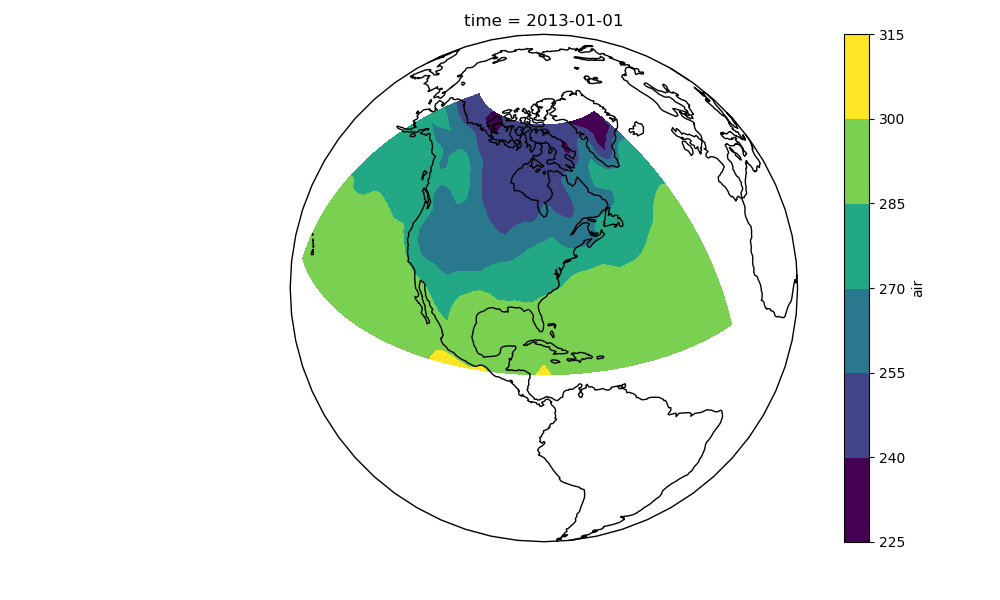When faceting on maps, the projection can be transferred to the plot function using the subplot_kws keyword. The axes for the subplots created by faceting are accessible in the object returned by plot:

In : p = air.isel(time=[0, 4]).plot(transform=ccrs.PlateCarree(), col='time',
....:                                subplot_kws={'projection': ccrs.Orthographic(-80, 35)})
....:

In : for ax in p.axes.flat:
....:     ax.coastlines()
....:     ax.gridlines()
....:

In : plt.show();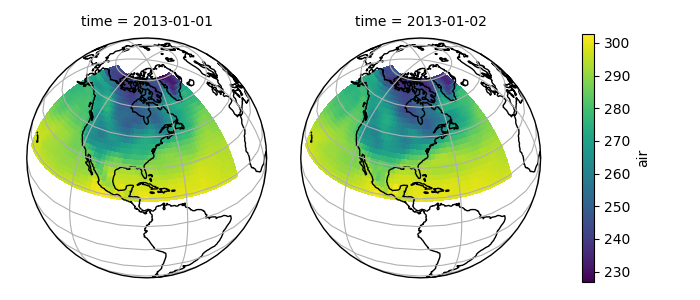## Details¶

### Ways to Use¶

There are three ways to use the xarray plotting functionality:

1. Use plot as a convenience method for a DataArray.
2. Access a specific plotting method from the plot attribute of a DataArray.
3. Directly from the xarray plot submodule.

These are provided for user convenience; they all call the same code.

In : import xarray.plot as xplt

In : da = xr.DataArray(range(5))

In : fig, axes = plt.subplots(ncols=2, nrows=2)

In : da.plot(ax=axes[0, 0])
Out: [<matplotlib.lines.Line2D at 0x7fa52f4db668>]

In : da.plot.line(ax=axes[0, 1])
Out: [<matplotlib.lines.Line2D at 0x7fa545147f98>]

In : xplt.plot(da, ax=axes[1, 0])
Out: [<matplotlib.lines.Line2D at 0x7fa52f4e20b8>]

In : xplt.line(da, ax=axes[1, 1])
Out: [<matplotlib.lines.Line2D at 0x7fa544cf1630>]

In : plt.tight_layout()

In : plt.show()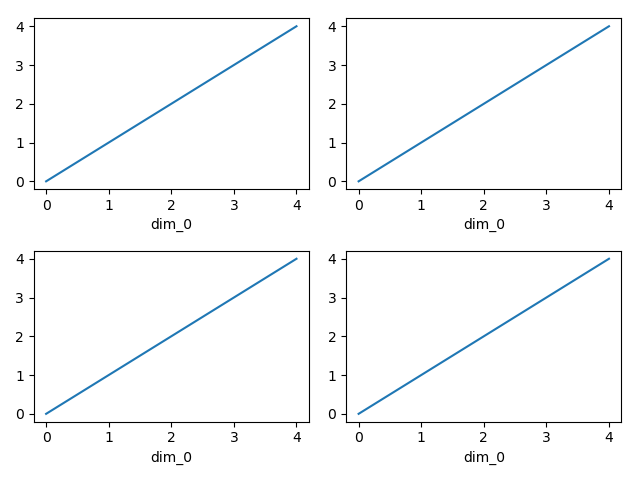Here the output is the same. Since the data is 1 dimensional the line plot was used.

The convenience method xarray.DataArray.plot() dispatches to an appropriate plotting function based on the dimensions of the DataArray and whether the coordinates are sorted and uniformly spaced. This table describes what gets plotted:

 Dimensions Plotting function 1 xarray.plot.line() 2 xarray.plot.pcolormesh() Anything else xarray.plot.hist()

### Coordinates¶

If you’d like to find out what’s really going on in the coordinate system, read on.

In : a0 = xr.DataArray(np.zeros((4, 3, 2)), dims=('y', 'x', 'z'),
....:                   name='temperature')
....:

In : a0[0, 0, 0] = 1

In : a = a0.isel(z=0)

In : a
Out:
<xarray.DataArray 'temperature' (y: 4, x: 3)>
array([[ 1.,  0.,  0.],
[ 0.,  0.,  0.],
[ 0.,  0.,  0.],
[ 0.,  0.,  0.]])
Dimensions without coordinates: y, x


The plot will produce an image corresponding to the values of the array. Hence the top left pixel will be a different color than the others. Before reading on, you may want to look at the coordinates and think carefully about what the limits, labels, and orientation for each of the axes should be.

In : a.plot()
Out: <matplotlib.collections.QuadMesh at 0x7fa52f406588>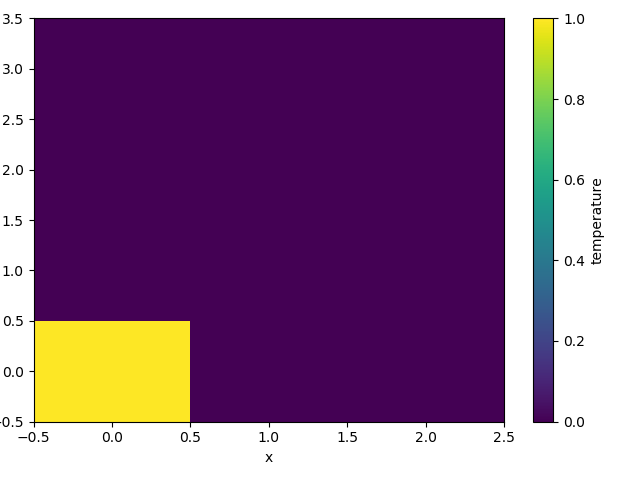It may seem strange that the values on the y axis are decreasing with -0.5 on the top. This is because the pixels are centered over their coordinates, and the axis labels and ranges correspond to the values of the coordinates.

### Multidimensional coordinates¶

You can plot irregular grids defined by multidimensional coordinates with xarray, but you’ll have to tell the plot function to use these coordinates instead of the default ones:

In : lon, lat = np.meshgrid(np.linspace(-20, 20, 5), np.linspace(0, 30, 4))

In : lon += lat/10

In : lat += lon/10

In : da = xr.DataArray(np.arange(20).reshape(4, 5), dims=['y', 'x'],
....:                   coords = {'lat': (('y', 'x'), lat),
....:                             'lon': (('y', 'x'), lon)})
....:

In : da.plot.pcolormesh('lon', 'lat');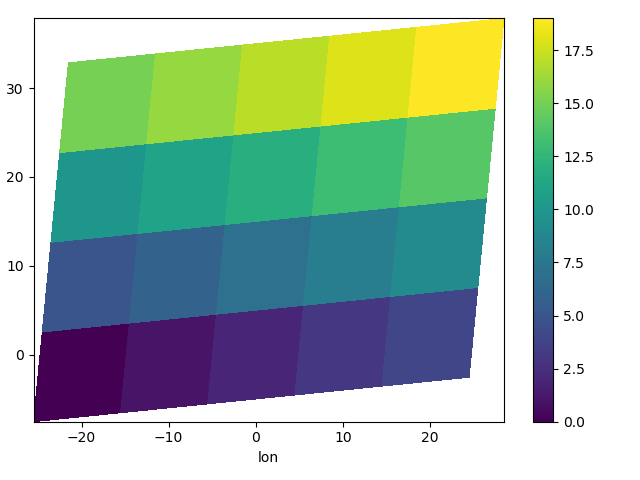Note that in this case, xarray still follows the pixel centered convention. This might be undesirable in some cases, for example when your data is defined on a polar projection (GH781). This is why the default is to not follow this convention when plotting on a map:

In : import cartopy.crs as ccrs

In : ax = plt.subplot(projection=ccrs.PlateCarree());

In : da.plot.pcolormesh('lon', 'lat', ax=ax);

In : ax.scatter(lon, lat, transform=ccrs.PlateCarree());

In : ax.coastlines(); ax.gridlines(draw_labels=True);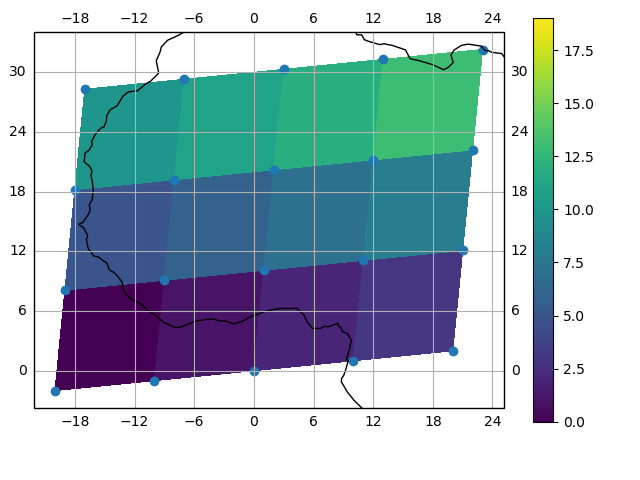You can however decide to infer the cell boundaries and use the infer_intervals keyword:

In : ax = plt.subplot(projection=ccrs.PlateCarree());

In : da.plot.pcolormesh('lon', 'lat', ax=ax, infer_intervals=True);

In : ax.scatter(lon, lat, transform=ccrs.PlateCarree());

In : ax.coastlines(); ax.gridlines(draw_labels=True);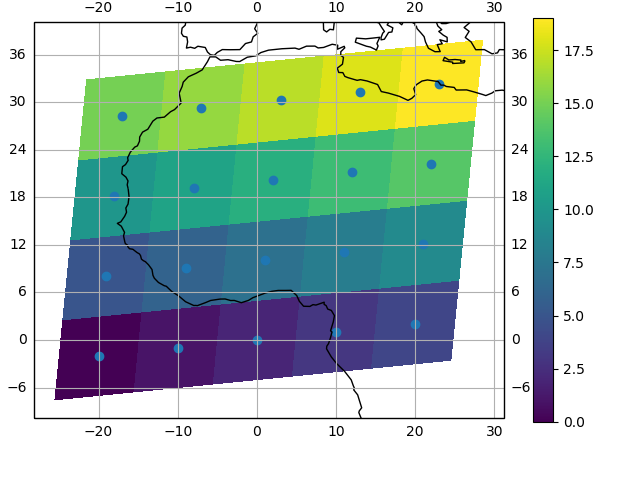Note

The data model of xarray does not support datasets with cell boundaries yet. If you want to use these coordinates, you’ll have to make the plots outside the xarray framework.